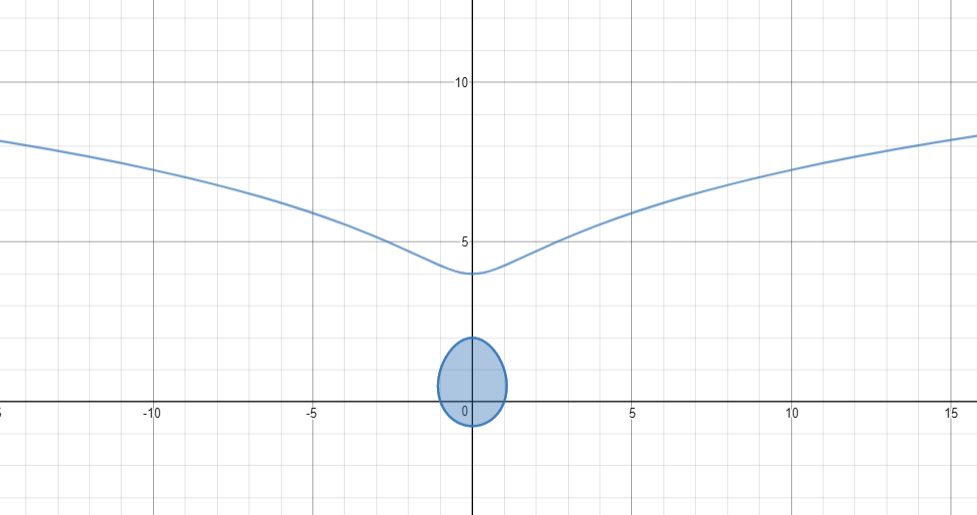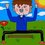# How does one find the area of this?Hello everyone! The relation of the image above is $x^2+y^2 = 2^y$. How do you find the area of the egg shape?

*Note: There is a section above the droplet, which should not be accounted for.

Made with Desmos graphing calculatorNote by Timothy Cao
3 years, 6 months ago

This discussion board is a place to discuss our Daily Challenges and the math and science related to those challenges. Explanations are more than just a solution — they should explain the steps and thinking strategies that you used to obtain the solution. Comments should further the discussion of math and science.

When posting on Brilliant:

• Use the emojis to react to an explanation, whether you're congratulating a job well done , or just really confused .
• Ask specific questions about the challenge or the steps in somebody's explanation. Well-posed questions can add a lot to the discussion, but posting "I don't understand!" doesn't help anyone.
• Try to contribute something new to the discussion, whether it is an extension, generalization or other idea related to the challenge.
• Stay on topic — we're all here to learn more about math and science, not to hear about your favorite get-rich-quick scheme or current world events.

MarkdownAppears as
*italics* or _italics_ italics
**bold** or __bold__ bold
- bulleted- list
• bulleted
• list
1. numbered2. list
1. numbered
2. list
Note: you must add a full line of space before and after lists for them to show up correctly
paragraph 1paragraph 2

paragraph 1

paragraph 2

[example link](https://brilliant.org)example link
> This is a quote
This is a quote
    # I indented these lines
# 4 spaces, and now they show
# up as a code block.

print "hello world"
# I indented these lines
# 4 spaces, and now they show
# up as a code block.

print "hello world"
MathAppears as
Remember to wrap math in $$ ... $$ or $ ... $ to ensure proper formatting.
2 \times 3 $2 \times 3$
2^{34} $2^{34}$
a_{i-1} $a_{i-1}$
\frac{2}{3} $\frac{2}{3}$
\sqrt{2} $\sqrt{2}$
\sum_{i=1}^3 $\sum_{i=1}^3$
\sin \theta $\sin \theta$
\boxed{123} $\boxed{123}$

## Comments

Sort by:

Top Newest

A different question about this graph: Consider graphs of the form $x^2+y^2=a^y$. If $a=2$, the graph is as above. If $a=2.09$, the egg shape is now connected to the upper segment of the graph but if $a=2.08$, the egg shape is still distinct. Therefore, at what value of $a$ does this egg first connect to the upper part of the graph?

- 3 years, 5 months ago

Log in to reply

Actually, that question has a precise answer: $a = e^{2/e} \approx 2.0871$.

$x^2 + y^2 = a^y.$

At the transition point, the tip of the "egg" and the dip in the rest of the graph meet each other; due to symmetry, this must happen somewhere on the line $x = 0$, and the connection will have a vertical tangent. Thus we set $dx = 0$ in the total derivative and substitute $a^y = y^2$:

$2x\:dx + 2y\:dy = (\ln a) a^y\:dy$ $2y = (\ln a) a^y = (\ln a)y^2.$ $\ln a = \frac 2 y\ \ \ \therefore\ \ \ a = e^{2/y}.$

Now substitute $x = 0$ and this result in the original equation: $y^2 = a^y = (e^{2/y})^y = e^2;$ $y = e,\ a = e^{2/e}.$

- 3 years, 5 months ago

Log in to reply

You can also generalize this solution for graphs of the form $x^{2n}+y^{2n} = a^y$. The general solution for the critical $a$ is $a = e^\frac{2n}{e}$

- 3 years, 5 months ago

Log in to reply

whfhbbjwhf

- 3 years, 5 months ago

Log in to reply

kjkjnklj

- 3 years, 5 months ago

Log in to reply

jnlkn

- 3 years, 5 months ago

Log in to reply

kn kjn

- 3 years, 5 months ago

Log in to reply

SVC

- 3 years, 5 months ago

Log in to reply

It is approximately 2.316, but I think you will have to resort to numerical integration as I did. Formally, we have $A = \int_{-c}^2 2\sqrt{2^y - y^2}dy,$ where $c \approx 0.767 > 0$ is such that $(\tfrac12)^c = c^2$; however, there is no hope to solve this integral algebraically.

- 3 years, 6 months ago

Log in to reply

×

Problem Loading...

Note Loading...

Set Loading...